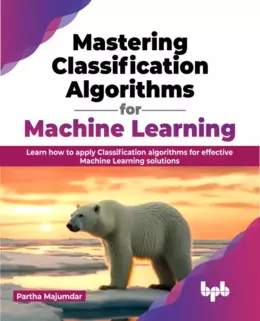# Mastering Classification Algorithms for Machine Learning### eBook Details:

• Paperback: 380 pages
• Publisher: WOW! eBook (May 23, 2023)
• Language: English
• ISBN-10: 935551851X
• ISBN-13: 978-9355518514

### Mastering Classification Algorithms for Machine Learning: Get familiar with all the state-of-the-art classification algorithms for machine learning

Classification algorithms are essential in machine learning as they allow us to make predictions about the class or category of an input by considering its features. These algorithms have a significant impact on multiple applications like spam filtering, sentiment analysis, image recognition, and fraud detection. If you want to expand your knowledge about classification algorithms, this book is the ideal resource for you.

The book starts with an introduction to problem-solving in machine learning and subsequently focuses on classification problems. It then explores the Naïve Bayes algorithm, a probabilistic method widely used in industrial applications. The application of Bayes Theorem and underlying assumptions in developing the Naïve Bayes algorithm for classification is also covered. Moving forward, the book centers its attention on the Logistic Regression algorithm, exploring the sigmoid function and its significance in binary classification. The book also covers Decision Trees and discusses the Gini Factor, Entropy, and their use in splitting trees and generating decision leaves. The Random Forest algorithm is also thoroughly explained as a cutting-edge method for classification (and regression). The book concludes by exploring practical applications such as Spam Detection, Customer Segmentation, Disease Classification, Malware Detection in JPEG and ELF Files, Emotion Analysis from Speech, and Image Classification.

• Learn how to apply Naïve Bayes algorithm to solve real-world classification problems
• Explore the concept of K-Nearest Neighbor algorithm for classification tasks
• Dive into the Logistic Regression algorithm for classification
• Explore techniques like Bagging and Random Forest to overcome the weaknesses of Decision Trees
• Learn how to combine multiple models to improve classification accuracy and robustness

By the end of the Mastering Classification Algorithms for Machine Learning book, you will become proficient in utilizing classification algorithms for solving complex machine learning problems.